# 深度学习word2vec学习笔记.pdf

by 北流浪子

2.1词向量

2.1.1 O

ne-Hot Representation

“话筒”表示为 [0 0 0 1 0 0 0 0 0 0 0 0 0 0 0 0 ...]

“麦克”表示为 [0 0 0 0 0 0 0 0 1 0 0 0 0 0 0 0 ...]

2.1.2 Distributed Representation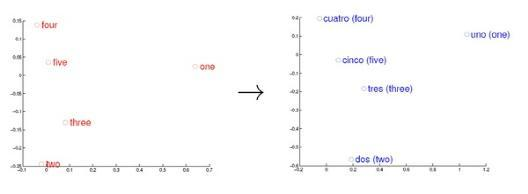2.2语言模型

2.2.1基本概念

ps=pw1,w2,⋯wT=pw1pw2w1)p(w3|w1,w2)⋯p(wt|w1,w2,⋯wT-1) (1)

P(大家，喜欢，吃，苹果)=p(大家)p(喜欢|大家)p(吃|大家,喜欢)p(苹果|大家,喜欢,吃)

p(大家)表示“大家”这个词在语料库里面出现的概率；

p(喜欢|大家)表示“喜欢”这个词出现在“大家”后面的概率;

p(吃|大家，喜欢)表示“吃”这个词出现在“大家喜欢”后面的概率；

p(苹果|大家,喜欢,吃)表示“苹果”这个词出现在“大家喜欢吃”后面的概率。

ps=pw1,w2,⋯wT=i=1Tp(wi|Contexti)

2.2.2 N-gram模型

pwiContexti=p(wi|wi-n+1,wi-n+2,⋯,wi-1)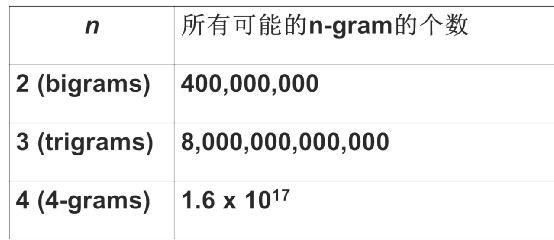N-gram模型也会有写问题，总结如下：

1、n不能取太大，取大了语料库经常不足，所以基本是用降级的方法

2、无法建模出词之间的相似度，就是有两个词经常出现在同一个context后面，但是模型是没法体现这个相似性的。

3、有些n元组（n个词的组合，跟顺序有关的）在语料库里面没有出现过，对应出来的条件概率就是0，这样一整句话的概率都是0了，这是不对的，解决的方法主要是两种：平滑法（基本上是分子分母都加一个数）和回退法（利用n-1的元组的概率去代替n元组的概率）

2.2.3N-pos模型

pwiContexti=pwi|c(wi-n+1),c(wi-n+2),⋯,c(wi-1)

2.2.4模型的问题与目标

L=i=1TpwiContexti

l=logL=1Vi=1TlogpwiContexti

L=jSij=1TjpwijContextij

l=logL=1Vj=1Sij=1TjlogpwijContextij

pwiContexti=f(wi,Contexti;θ)

 http://techblog.youdao.com/?p=915 Deep Learning实战之word2vec，网易有道的pdf

 第五章 n-gram语言模型 百度文库上的一个资料

 主题：统计自然语言处理的数学基础 百度文库上的一个资料

Word2vec总共有两种类型，每种类型有两个策略，总共4种。这里先说最常用的一种。这种的网络结构如下图。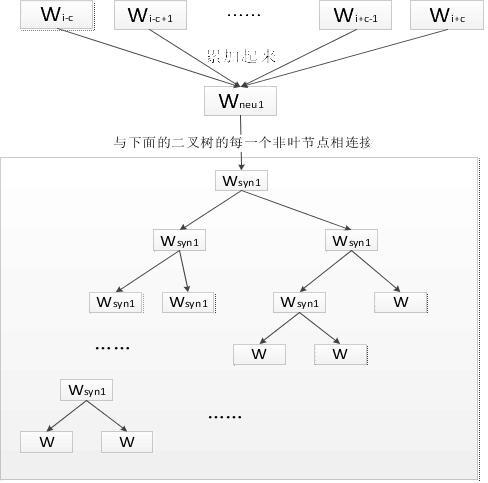ps=pw1,w2,⋯wT=i=1Tp(wi|Contexti)

p吃Context吃=(1-σA∙C)∙(1-σB∙C)

p吃Context吃=(1-σA∙C)∙σB∙C∙(1-σE∙C)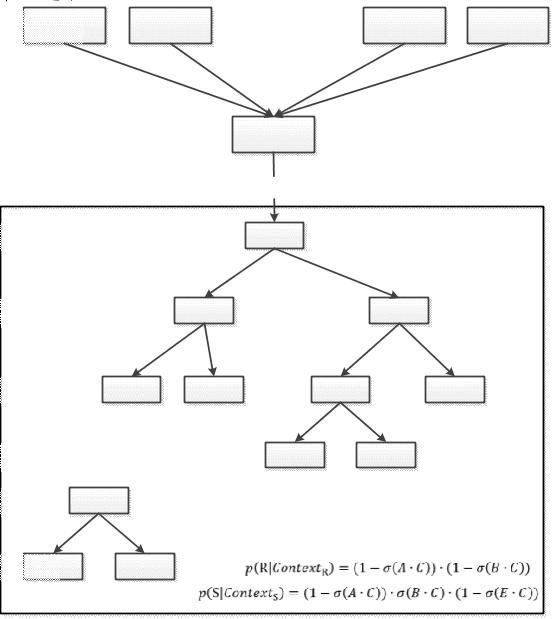2.1从霍夫曼树到条件概率的计算

EA,C=-(A∙C)

pAC=e-E(A,C)v=1Ve-E(wv,C) (2.1.2)

pAC=p(A|G,C)p(G|C) (2.1.3)

PY=yX=x=P(Y=y|D=dy,X)P(D=d(y)|X=x)

PYX=iPY,D=iX=iPYD=i,XPD=iX =P(Y|D=dY,X)P(D=d(Y)|X)

pGC=e-E(G,C)e-E(G,C)+e-E(H,C)=11+e-(-(H-G)∙C)=11+e-E(H-G,C)

pAG,C=e-E(A,C)W∈Ge-E(W,C)

pAG,C=p(A|GH,G,C)p(GH|G,C)

pGHG,C=11+e-E(GG-GH,C)

pAGH,G,C=e-E(A,C)W∈GHe-E(W,C)

pAC=p(A|GH,G,C)p(GH|G,C)p(G|C)

pAC=pAGHGG,GHG,GH,G,Cp(GHGG|GHG,GH,G,C)p(GHG|GH,G,C)p(GH|G,C)p(G|C)

pAC=p(GHGG|GHG,GH,G,C)p(GHG|GH,G,C)p(GH|G,C)p(G|C)

pAC=11+e-E(GHH-GHG,C)∙11+e-E(GG-GH,C)∙11+e-E(H-G,C)

pHC=11+e-E(F,C)

pwContext=k=1Kp(dk|qk,C)=k=1Kσ(qk∙C)1-dk∙(1-σ(qk∙C))dk

2.1.1霍夫曼树

2.2目标函数

L(θ)=jSij=1TjpwijContextij
(2.2.1)

l(θ)=logL(θ)=1Vj=1Sij=1TjlogpwijContextij
(2.2.2)

Lθ=jSij=1TjpwijContextij=jSij=1Tjkij=1Kijσ(qkij∙Cij)1-dkij∙1-σ(qkij∙Cij)dkij

lθ=logLθ=j=1Sij=1Tjkij=1Kijlogσqkij∙Cij1-dkij∙1-σqkij∙Cijdkij=j=1Sij=1Tjkij=1Kij1-dkijlogσqkij∙Cij+dkijlog1-σqkij∙Cij

2.3解法

fij=pwijCij=-kij=1Kij1-dkijlogσqkij∙Cij+dkijlog1-σqkij∙Cij

fkij=-1-dkijlogσqkij∙Cij-dkijlog1-σqkij∙Cij

Fqqkij=∂fkij∂qkij=-1-dkij∙1-σqkij∙Cij∙Cij-dkij∙-σqkij∙Cij∙Cij=-1-dkij-σqkij∙Cij∙Cij

Fcqkij=∂fkij∂Cij=-1-dkij∙1-σqkij∙Cij∙qkij-dkij∙-σqkij∙Cij∙qkij=-1-dkij-σqkij∙Cij∙qkij

qkijn+1=qkijn-ηFqqkijn

wIn+1=wIn-ηkij=1KijFcqkijn

2.4代码中的trick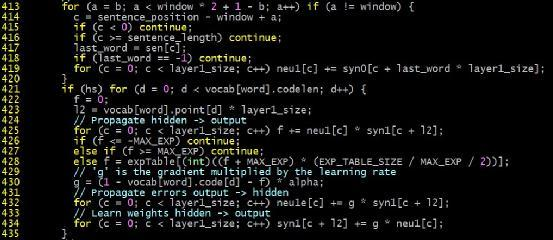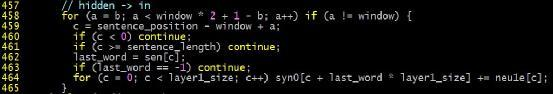3.1网络结构与使用说明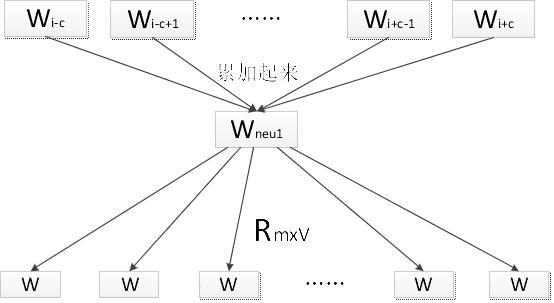p吃Context吃=σ(A∙C)∙1-σ(D∙C)∙1-σ(E∙C)∙1-σ(F∙C)

4.1抽样方法的意义与目标函数

logσwij∙Cij+k=1KEwk~pV(w)log1-σwk∙Cij

logσwij∙Cij+k=1Klog1-σwk∙Cij

fw=-label∙logσw∙Cij-(1-label)log1-σw∙Cij

4.2CBOW加抽样方法的解法

Fw(w)=∂fw∂w=-label∙1-σw∙Cij∙Cij+1-label∙σw∙Cij∙Cij=-label-σw∙Cij∙Cij

Fc(w)=∂fw∂Cij=-label∙1-σw∙Cij∙w+1-label∙σw∙Cij∙w=-label-σw∙Cij∙w

Rwn+1=Rwn-ηFw(Rwn)

wIn+1=wIn-ηFcRwIn+k=1KFcRwkn

4.3CBOW加抽样方法代码中的trick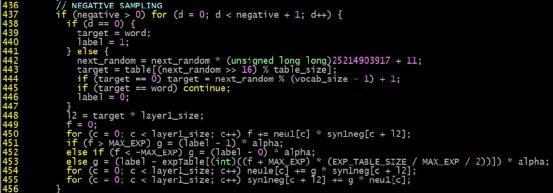5.1网络结构与使用说明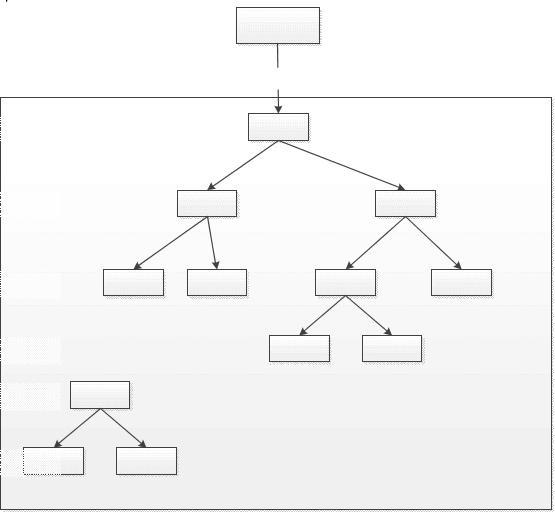p大家吃=(1-σA∙D)∙σB∙D∙σC∙D

5.2目标函数

L(θ)=jSij=1Tj-cij

ij=1Tj-cij

l(θ)=logL(θ)=1Vj=1Sij=1Tj-cij (5.2.2)

pwI=k=1Kp(dk|qk,I)=k=1Kσ(qk∙I)1-dk∙(1-σ(qk∙I))dk

logpwI=k=1K1-dklogσqk∙I+dk∙1-σ(qk∙I)

5.3解法

lf=pdkqk,I=-1-dklogσqk∙I-dk∙1-σ(qk∙I)

Fqqk=∂lf∂qk=-1-dk∙1-σqk∙I∙I-dk∙-σqk∙I∙I=-1-dk-σqk∙I∙I

Fiqk=∂lf∂I=-1-dk∙1-σqk∙I∙qk-dk∙-σqk∙I∙qk=-1-dk-σqk∙I∙qk

qkn+1=qkn-ηFq(qkn)

In+1=In-ηFi(qkn)

5.4代码中的trick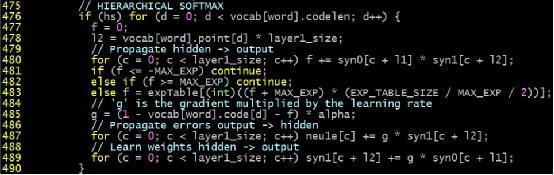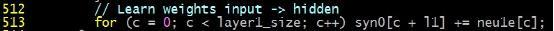6.1网络结构与使用说明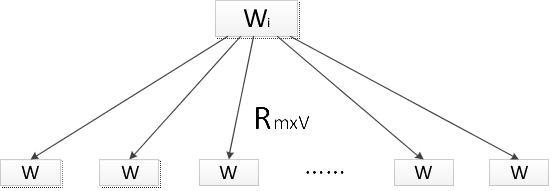p吃Context吃=σ(A∙C)∙1-σ(D∙C)∙1-σ(E∙C)∙1-σ(F∙C)

6.2目标函数与解法

logσw∙I+k=1KEw~pV(w)log1-σwk∙Cij

fw=-label∙logσw∙I-(1-label)log1-σw∙I

Fw(w)=∂fw∂w=-label∙1-σw∙I∙Cij+1-label∙σw∙I∙I=-label-σw∙I∙I

Fc(w)=∂fw∂I=-label∙1-σw∙I∙w+1-label∙σw∙I∙w=-label-σw∙I∙w

Rwn+1=Rwn-ηFw(Rwn)

In+1=In-ηFcRInn+k=1KFcRwkn

6.3代码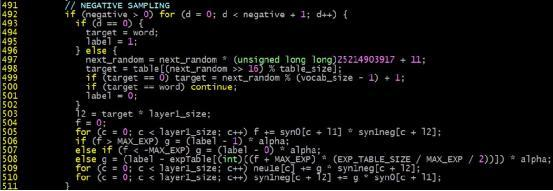http://techblog.youdao.com/?p=915 Deep Learning实战之word2vec，网易有道的pdf

 http://xiaoquanzi.net/?p=156 hisen博客的博文

 Hierarchical probabilistic neural network language model. Frederic Morin and Yoshua Bengio.

 Distributed Representations of Words and Phrases and their Compositionality T. Mikolov, I. Sutskever, K. Chen, G. Corrado, and J. Dean.

 A neural probabilistic language model Y. Bengio, R. Ducharme, P. Vincent.

 Linguistic Regularities in Continuous Space Word Representations. Tomas Mikolov,Wen-tau Yih,Geoffrey Zweig

 Efficient Estimation of Word Representations in Vector Space. Tomas Mikolov,Kai Chen,Greg Corrado,Jeffrey Dean.

1.1基本概念

1.2兴趣挖掘的必要性

1.3利用word2vec给广告主推荐用户

U1 a1,a2,a3……

U2 a2,a3,a5,……

U3 a1,a3,a6,……

a1,a2,a3……

a2,a3,a5,……

a1,a3,a6,……

A1 (0.3,-0.5,0.1)

A2 (0.1,0.4,0.2)

A3 (-0.3,0.7,0.8)

……

An (0.7,-0.1,0.3)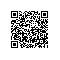使用钉钉扫一扫加入圈子
+ 订阅